# Regular 5-gon

Calculate the area of the regular pentagon with side 7 cm.

S =  84.3 cm2

### Step-by-step explanation: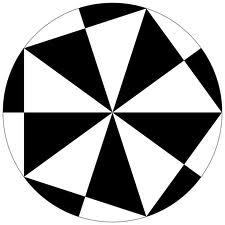Did you find an error or inaccuracy? Feel free to write us. Thank you!Tips to related online calculators

#### You need to know the following knowledge to solve this word math problem:

We encourage you to watch this tutorial video on this math problem:

## Related math problems and questions:

• PentagonCalculate the area of a regular pentagon, which diagonal is u=16.
• n-gon IIWhat is the side length of the regular 5-gon circumscribed circle of radius 11 cm?
• n-gonWhat is the side length of the regular 5-gon inscribed in a circle of radius 12 cm?
• Nine-gonCalculate the perimeter of a regular nonagon (9-gon) inscribed in a circle with a radius 13 cm.
• PentagonCalculate the length of side, circumference and area of a regular pentagon, which is inscribed in a circle with radius r = 6 cm.
• Regular n-gonWhich regular polygon have a radius of circumscribed circle r = 10 cm and the radius of inscribed circle p = 9.962 cm?
• Hexagon ACalculate area of regular hexagon inscribed in circle with radius r=9 cm.
• Inscribed and described circle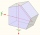Find the radii of a circle inscribed and circumscribed by a regular pentagon whose side measures 3 cm.
• Find the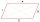Find the content of a regular 12 sided polygon if its side a = 12 cm.
• Right triangle trigonometricsCalculate the size of the remaining sides and angles of a right triangle ABC if it is given: b = 10 cm; c = 20 cm; angle alpha = 60° and the angle beta = 30° (use the Pythagorean theorem and functions sine, cosine, tangent, cotangent)Calculate the content of a regular 15-sides polygon inscribed in a circle with radius r = 4. Express the result to two decimal places.How many glass are needed to produce glass with base regular 5-gon if one base triangle in the base is 4.2 square cm and the height is 10 cm?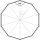Which of the following numbers most accurately area of a regular decagon with side s = 2 cm? (A) 9.51 cm2 (B) 20 cm2 (C) 30.78 cm2 (D) 31.84 cm2 (E) 32.90 cm2Calculate interior angles of the isosceles triangle with base 40 cm and legs 22 cm long.Calculate the missing side b and interior angles, perimeter, and area of ​​a right triangle if a=10 cm and hypotenuse c = 16 cm.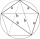Calculate the side a, the circumference and the area of the regular 5-angle if Rop = 6cm.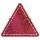Calculate the inradius and circumradius of an equilateral triangle with side a=77 cm.